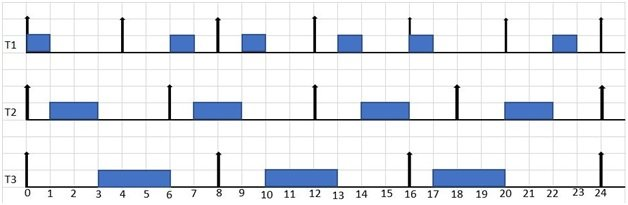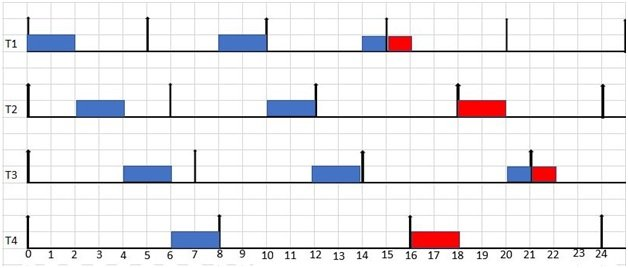# EARLIEST DEADLINE FIRST (EDF) SCHEDULING ALGORITHM

### EARLIEST DEADLINE FIRST (EDF) SCHEDULING ALGORITHM

EDF is an optimal algorithm which means if a task set is feasible then it is surely scheduled by EDF. Another thing is that EDF does not specifically take any assumption on periodicity of tasks so it is independent of Period of task and therefore can be used to schedule aperiodic tasks as well. If two tasks have same absolute deadline choose one of them randomly. you may also like to read

### Example of EARLIEST DEADLINE FIRST (EDF) SCHEDULING ALGORITHM

An example of EDF is given below for task set of table-2.

 Task Release time(ri) Execution Time(Ci) Deadline (Di) Time Period(Ti) T1 0 1 4 4 T2 0 2 6 6 T3 0 3 8 8

U= 1/4 +2/6 +3/8 = 0.25 + 0.333 +0.375 = 0.95 = 95%

As processor utilization is less than 1 or 100% so task set is surely schedulable by EDF.Figure 2. Earliest deadline first scheduling of task set in Table -2

1. At t=0 all the tasks are released, but priorities are decided according to their absolute deadlines so T1 has higher priority as its deadline is 4 earlier than T2 whose deadline is 6 and T3 whose deadline is 8, that’s why it executes first.
2. At t=1 again absolute deadlines are compared and T2 has shorter deadline so it executes and after that T3 starts execution but at t=4 T1 comes in the system and deadlines are compared, at this instant both T1 and T3 has same deadlines so ties are broken randomly so we continue to execute T3.
3. At t=6 T2 is released, now deadline of T1 is earliest than T2 so it starts execution and after that T2 begins to execute. At t=8 again T1 and T2 have same deadlines i.e. t=16, so ties are broken randomly an T2 continues its execution and then T1 completes. Now at t=12 T1 and T2 come in the system simultaneously so by comparing absolute deadlines, T1 and T2 has same deadlines therefore ties broken randomly and we continue to execute T3.
4. At t=13 T1 begins it execution and ends at t=14. Now T2 is the only task in the system so it completes it execution.
5. At t=16 T1 and T2 are released together, priorities are decided according to absolute deadlines so T1 execute first as its deadline is t=20 and T3’s deadline is t=24.After T1 completion T3 starts and reaches at t=17 where T2 comes in the system now by deadline comparison both have same deadline t=24 so ties broken randomly ant we T continue to execute T3.
6. At t=20 both T1 and T2 are in the system and both have same deadline t=24 so again ties broken randomly and T2 executes. After that T1 completes it execution. In the same way system continue to run without any problem by following EDF algorithm.

### Transient Over Load Condition & Domino Effect in Earliest deadline first

 Task Release time(ri) Execution Time(Ci) Deadline (Di) Period(Ti) T1 0 2 5 5 T2 0 2 6 6 T3 0 2 7 7 T4 0 2 8 8Figure 3. Domino effect under Earliest deadline first

As in the above figure at t=15 T1 misses it deadline and after that at t=16 T4 is missing its deadline then T2 and finally T3 so the whole system is collapsed. It is clearly proved that EDF has a shortcoming due to domino effect and as a result critical tasks may miss their deadlines. The solution of this problem is another scheduling algorithm that is least laxity first (LLF). It is an optimal scheduling algorithm. Demand bound function ad Demand bound analysis are also used for schedualability analysis of given set of tasks.

### Advantages of EDF over rate monotonic

• No need to define priorities offline
• It has less context switching than rate monotonic
• It utilize the processor maximum up to 100% utilization factor as compared to rate monotonic

### Disadvantages of EDF over rate monotonic

• It is less predictable. Because response time of tasks are variable and response time of tasks are constant in case of rate monotonic or fixed priority algorithm.
• EDF provided less control over the execution